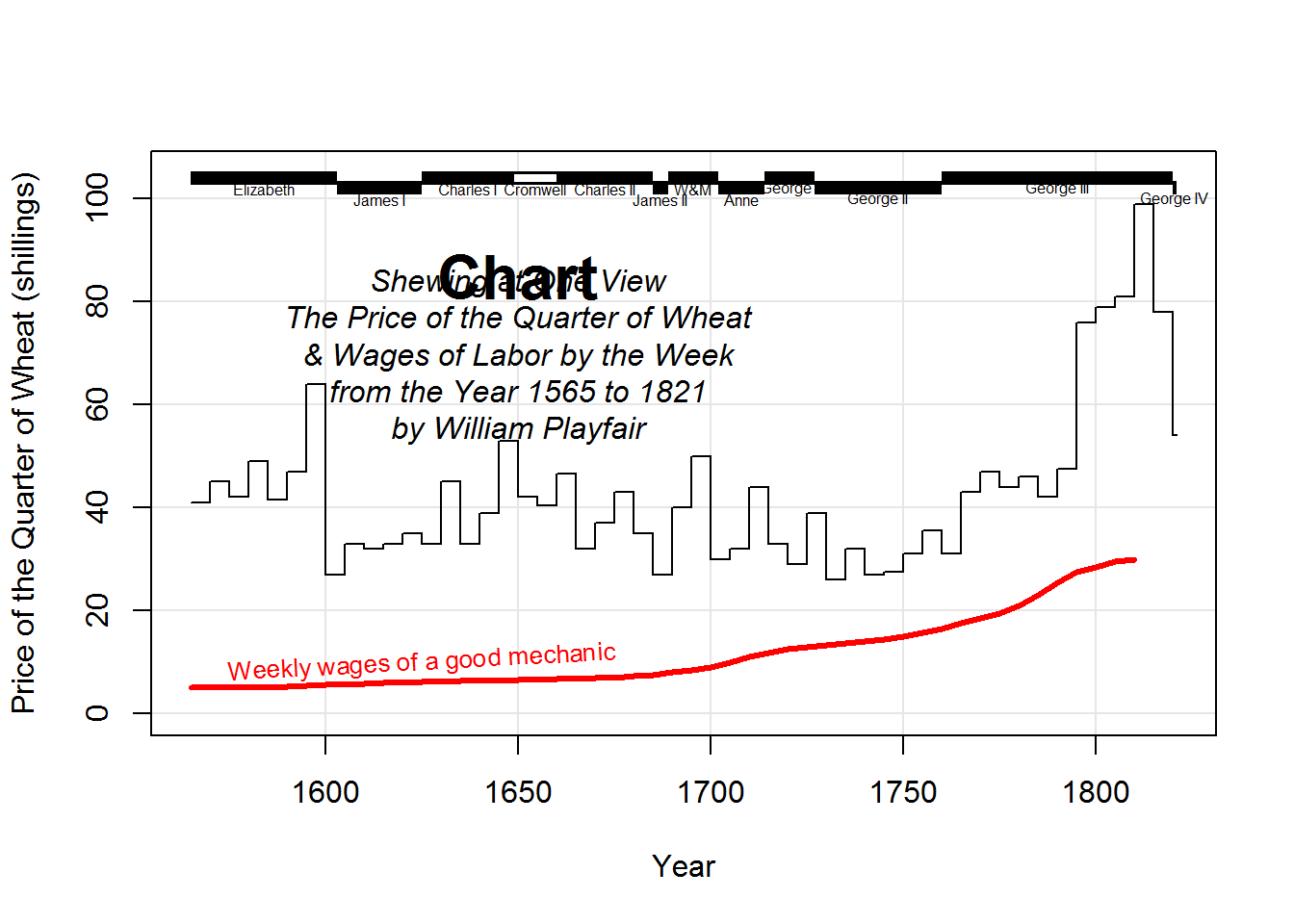data(Wheat, package="HistData")
data(Wheat.monarchs, package="HistData")

# For best results, this graph should be viewed with width ~ 2 * height
# Note use of type='s' to plot a step function for Wheat
# and panel.first to provide a background grid()
# The curve for Wages is plotted after the polygon below it is filled
with(Wheat, {
plot(Year, Wheat, type="s", ylim=c(0,105),
ylab="Price of the Quarter of Wheat (shillings)",
panel.first=grid(col=gray(.9), lty=1))
lines(Year, Wages, lwd=3, col="red")
})

# add some annotations
text(1625,10, "Weekly wages of a good mechanic", cex=0.8, srt=3, col="red")

# cartouche
text(1650, 85, "Chart", cex=2, font=2)
text(1650, 70,
paste("Shewing at One View",
"The Price of the Quarter of Wheat",
"& Wages of Labor by the Week",
"from the Year 1565 to 1821",
"by William Playfair",
sep="\n"), font=3)

# add the time series bars to show reigning monarchs
# distinguish Cromwell visually, as Playfair did
with(Wheat.monarchs, {
y <- ifelse( !commonwealth & (!seq_along(start) %% 2), 102, 104)
segments(start, y, end, y, col="black", lwd=7, lend=1)
segments(start, y, end, y, col=ifelse(commonwealth, "white", NA), lwd=4, lend=1)
text((start+end)/2, y-2, name, cex=0.5)
})